# Data Science

Read the latest articles about Data Science at Infosimples.com

•TensorFlow is the Deep Learning library with the most growth potential
•A little about how Shazam works
•Unsupervised learning shows which senators vote alike
•What is Data Matching?
•Semantic Analytics with Google Cloud Dataflow (webinar)
•Data engineering over the Titanic disaster
•Deep Learning Webinar promoted by NVIDIA
•Overview of Neural Networks
•Artificial Intelligence for Tic-Tac-Toe
•String Segmentation using Machine Learning

TensorFlow is the Deep Learning library with the most growth potential

Deep Learning libraries have gained popularity in Machine Learning applications. Here are the leading ones:

Library Support Top users
Caffe C++, Python NVIDIA, general industry
SINGA C++, Python Apache
Theano Python Yoshua Bengio (University of Montreal),
some researchers at Google
Torch C, Lua Yann LeCun (Facebook)

I'll not get into details on each one. It's worth mentioning that Theano and Torch are mostly used in cientific research, Caffe is mostly used in commercial Machine Learning applications and SINGA was launched recently by the Apache Foundation and seems to compete mostly with Caffe.

At Infosimples we mostly use Caffe because it has great support for processing images. Any of these libraries is a great choice and they have great communities with active development.

A new options was introduced yesterday and that's why I've decided to write this mini article. I'm talking about TensorFlow, developed by a group of renowned researchers at Google. The researchers themselves are a top reason to call our attention. They have published great articles in Machine Learning conferences and some of them have contributed to the development of the libraries I mentioned in the table above.

In addition to being flexible to be used by the cientific community, TensorFlow is also a great choice to deploy commercial applications of Machine Learning, with great support to run in servers, desktops and mobile devices.

I think this tool have everything in place to become a leading resource in Machine Learning development. So, if you want to get into this field, now is a great time. I recommend you to begin with the tutorials of TensorFlow.

I hope to bring more news soon. I'll leave the TensorFlow launch video below.

A little about how Shazam works

## Have you ever heard of Shazam?

Shazam is a very popular mobile app that allows you to recognize almost any song playing at your surroundings. You may be on a book store, listening to the beats of a soothing jazz or at your favorite American-style coffee shop, where the Jukebox rocks a contagious but unknown country-style music. Just pop the app, record a small piece of music and Shazam will tell you its name, its artist and where to buy it. Booya!##### Just give it some seconds to guess (almost) any music.

In this article, which was inspired and based on this readings, I'll try explain the basics of its working. How it knows so much? And how it does it so fast?

## How does a computer see a music?

Physically, a piece of sound is a mechanic wave, which goes through the air in different heights and frequencies causing our tympanums to vibrate. Visually, it can be represented by a messy scrawl, with peaks and lows. If you ever played with Windows’ Sound Recorder you may know what I'm talking about.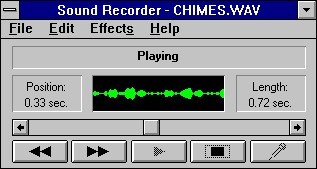#### When the computer records a sound, it does it by taking samples, in identical intervals, of the height of that continuous wave, generating a plot full of dots: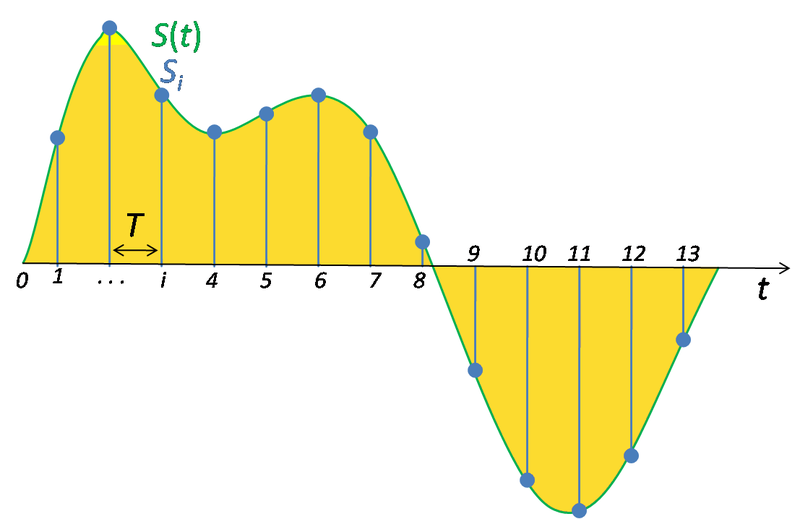Example of how a computer samples a sound. T is the interval between each sample.

If those dots are close enough to each other, the computer will have an easy time recomposing it. If that happens, you can imagine that they are so squeezed together that they look almost identical to the original wave. Therefore, the higher the sample rate (i.e.: the lower the time interval), the closer the digital sound is to the original sound. In other words, a good sampling rate provides a clear sound.

To give you an idea, an usual music CD stores its audio at 44100 samples per second (44,1kHz) and so does the majority of the music you listen on your smartphone or MP3 player. A DVD stores its audio files at more than double this rate (96kHz). Audiophiles claim that crystal-clear music is only possible to achieve at 192kHz.

Besides sound quality, an acceptable sample rate is important because the computer needs to reconstruct the continuous signal correctly: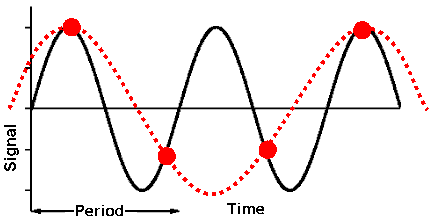The image above is an example of a incorrectly reconstructed wave. Notice how the recomposed signal (dashed in red) is different from the original sound (in black). This happens beacuse two samples are too far from one another, and the computer connects the dots in a wrong way. According to the Nyquist rule, - and its not too hard to see - the sample rate needs to be at least twice as the original wave frequency so the wave can be ‘redrawed’ with no flaws.

## A brief explanation on sines, cosines and complex numbers

Sinusoids and complex numbers are very important when dealing with any kind of signal. I’ll try to simplify some things, so a high-school knowledge must be sufficient to get an idea on what’s going on. Here’s a resume of the most important concepts:

Sines and cosines:
A sinusoidal wave can be written as it follows:

$$f(t) = D + Asin(k + \omega t)$$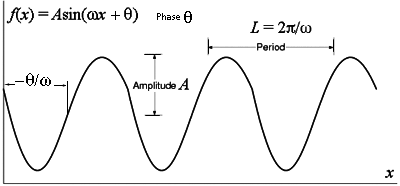A sinusoid with all its parameters.

Keep in mind that the most important parameters in this function are the amplitude A and the angular frequency $$\omega$$, since they permanently change the forms of the wave and tell us how it behaves along time, whereas D and k just deslocate it through the graph. 'A' tells how high the wave can go and $$\omega$$ tells how fast the wave goes through the time axis. If you have both of them, you can completely describe the behavior of a waveform.

Complex numbers

Complex numbers are an auxiliary tool in mathematics. In high school, most of you must have some hard time to find a utility to it. Although they don’t make any sense in real life, their properties can make mathematical operations a lot easier. They are very useful, for example, in Electrical Engineering, where we are constantly dealing with tensions, currents and much other periodic elements.

The most important sine and cosine parameters can be synthetized on a formula envolving the imaginary unit i as it foilows: $$f(t) = Ae^{i \omega t}$$. This may be unfamilliar for those who never studied complex numbers a little deeper. What does it mean to take a real number to the power of the imaginary unit?

As a result of the expansion of a MacLaurin series, from Calculus, it is possible to show that sines and cosines can be written using mixins of Euler unit to the power of i. If you want to go deeper, you can take a look at this webblog, (units 1 to 3) but for the understanding of this article it is sufficient to know that the main properties of a sinusoid can be condensed on the 'complex way' as $$f(t) = Ae^{i \omega t}$$, since it contains both A and $$\omega$$. $$f(t)$$ is also equal to the much more high-school familliar $$A(cos(\omega t) + isen(\omega t))$$, where we just made the angle $$(\text{usually } \theta)$$ time variable $$(\omega t)$$.

With this in mind, we can show a different way to describe those functions, with a graph just for 'A' and 'w'. We’ll soon see the reason for this.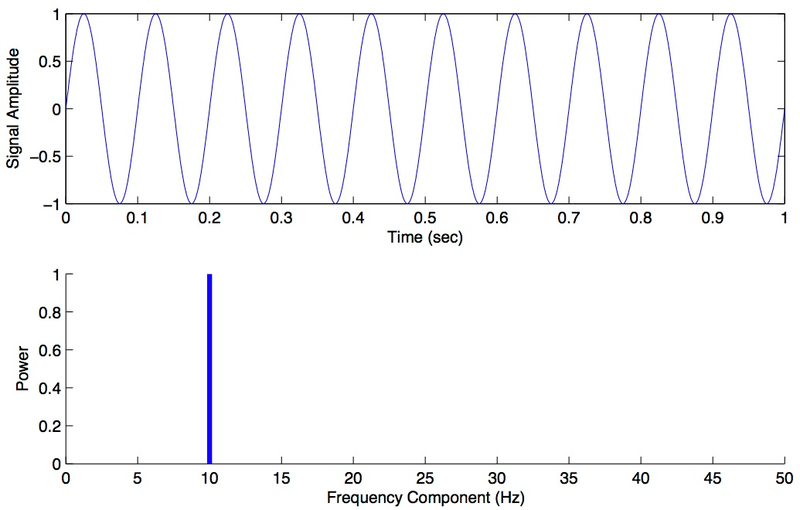##### Above, $$f(t) = sin(20 \pi t)$$. Notice A = 1 and $$\omega = \frac{20 \pi}{2 \pi} = 10$$. Below $$f(t), F(w)$$, where we just explicit A and $$\omega$$.

By doing this, we say that we are taking this function from the time domain to the frequency domain. This is very intuitive for simple sinewaves, but it will get a little more complex for other waveforms. Hopefully, there is a mathematical operation that takes us from one domain to another. It's called the Fourier Transform:

$$F(\omega) = \int_{-\infty}^\infty f(t){e}^{-i \omega t}\,\mathrm{d}t$$

f(t) denotes the time domain function and F(w) the frequency domain function. There is no need to understand integrals here, just take in mind that there is an algebraheic way to obtain the frequency function from the time function (and vice-versa - using the Inverse Fourier Transform).

Decomposing scrawls into sinewaves

To ease our understandings, lets strategically add some sinusoids with different frequencies and amplitudes with the help of a computer graphing software. Don't worry about the craziness of the function we chose, just take a look of its plot: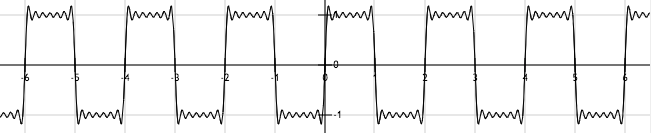$$f(t) = \frac {4} {\pi}[sin(\pi t) + \frac {sin(3 \pi t)}{3}+ \frac {sin(5 \pi t)}{5}+ \frac {sin(7 \pi t)}{7}+ \frac {sin(9 \pi t)}{9}+ \frac {sin(11 \pi t)}{11}]$$

Note how this resembles the square wave:

####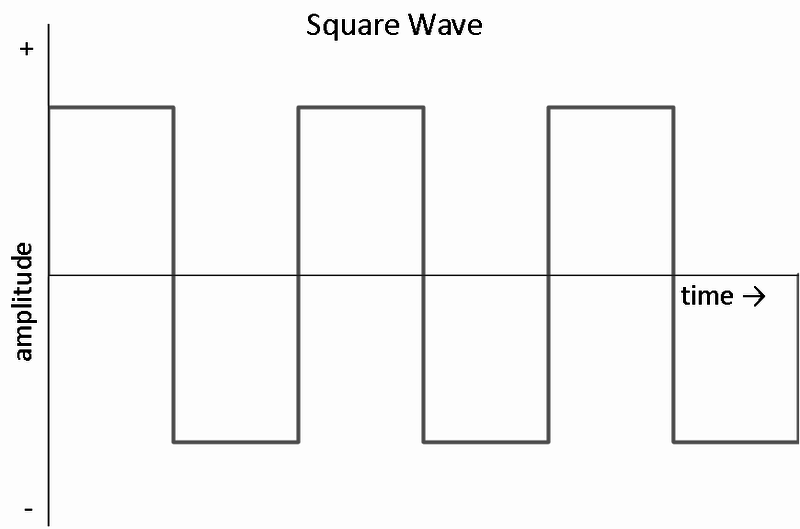Actually, if we keep infinitely adding sinusoids with this pattern we'll end up exactly with the square wave: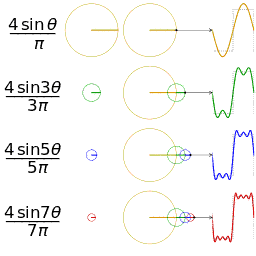Cool, huh?

The point here is we can achieve very cool waveforms just by adding sinewaves. In fact, if we do it correctly, we can achieve any periodic waveform just by adding sinewaves.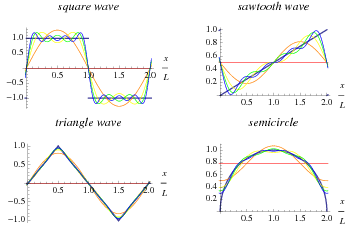Any waveform (even crazy ones) can be written as a sum of sines and cosines!

This decomposing is useful simply because trigonometric functions are very easy to work with.

Although, it may be a little messy to describe the wave on the time domain as a summation of so many different amplitudes and frequencies. There's where the Fourier Transform becomes handy. As an example, look how a waveform composed of 6 sinewaves (in red) can be easily represented on the frequency domain (in blue). Both domains contain the same information, but the frequency domain presents it on a much cleaner way.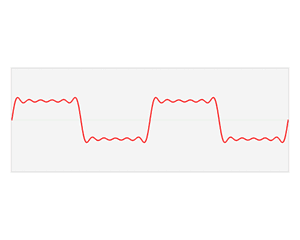## Back to Shazam

Shazam compares the piece of sound you recorded to the different musics it has on its databases and returns the music that has the greatest maching ratio. It may sound simple, but take in mind that Shazam has data of over 11 million songs on its servers. It would take a very long time for the search to succeed if this comparison wasn't optimized.

That known, we need to generate some elements from our sound record that can be rapidly compared to the elements on Shazam's server side, which were generated on the same way. We'll call these elements fingerprints.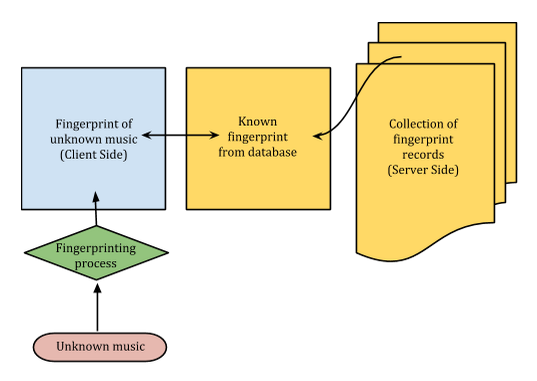A litte schema on how Shazam does its jobs

Some paragraphs above, we discussed how a waveform can be decomposed into sinewaves, which can be written in terms of its frequencies just by applying the Fourier Transform on it. Suppose you want to do it on your music record just to see what you get.

On the stepway, you'll see there's a problem. Remember how the waveform seen by a computer is a plot full of dots instead of a continuous curve? For that reason you cannot apply the Fourier Transform as described above, since the integral operation isn't defined for non-continuous functions.

Because of that, instead using the 'conventional' Fourier transform, we'll use its discrete counterpart, called Discrete Fourier Transform, or simply DFT, whose formula is: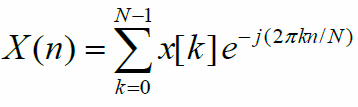Where:

- $$X(n)$$ is the frequency domain of the n-th bin

- $$N$$ is the window size

- $$x[k]$$ is the time domain wave function you want to transform

Notice some strange parameters appeared here. That's because we are going to apply the DFT several times on consecutive parts of the soundwave and not on the entire wave at once, as we did on the first example. This is to obtain the different frequency spectrums at each small period of time, which we will call chunk. In other words, we'll obtain lots of Amplitude x Frequency graphs (X(n)), one for each chunk.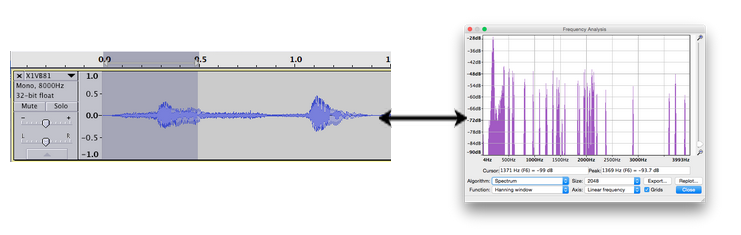Here we highlighted a chunk with 0.5s of sound and its frequency spectrum. There will be a pair of these each 0.5s of music. Notice we have multiple different frequencies here, differently from our upper example

The parameter that defines the length of this chunk is the upper case N and is called Window Size. The greater the window size (i.e.: the greater the length of the waveform captured), the larger our chunks will be and the less the number of Amplitude x Frequency graphs (frequency spectrums) will be generated.

A window, whose size is a mandatory power of 2, mustn't be either too high or low to correctly generate the spectrums. A high window size provides a low number of spectrum samples, but is more accurate on determining their frequencies. The opposite is valid for low window sizes. A good window size is between 1024 and 4096.

This operations can be easily done on a computer with help of Ruby or Python math modules. Further, we'll take some time optimizing some algorithms to do the calculations faster

What do you mean by 'accurate'?

On the last paragraph I mentioned about accuracy when windowing a sound, saying wider windows provides greater exactitude to frequencies. To understand that, we'll go a little deeper into Fourier transforms.

For understanding purposes, let's assume the piece of sound you recorded is a continuous wave. As we saw, we need to take the Fourier Transform on a part of the piece of sound you recorded. As we are assuming the sound is continuous, we can multiply the function (i.e.: the sound) by a Heaviside function to make all its extention equals 0 except on the desired part. We can then, apply the original Fourier Transform on it.

Note: If it still sounds confusing, I recommend you spending some minutes reading the Heaviside function article. It’s basics are very easy to understand.

Denoting the piece of sound by $$S(t)$$, the Heaviside function by $$H(t)$$, the part of the piece of sound by $$PartOfS(t)$$ and the Fourier Transform by $$\mathcal{F}$$, we have:

$$PartOfS(t) = S(t) x H(t)$$

Applying the Fourier Transform on both sides:

$$\mathcal{F}[PartOfS(t)] = \mathcal{F}[S(t) x H(t)]$$

As of the convolution theorem:

$$\mathcal{F}[PartOfS(t)] = \mathcal{F}[S(t)] \bigotimes \mathcal{F}[H(t)]$$

The convolution is a mathematical operation just like the multiplication or the division, but there’s no need to understand it deeply. The point here is to show that the result of the Fourier Transform will be dependent on the function that multiplies the $$S(t)$$. If we happen to choose any different function than Heaviside's the result of the Fourier Transform will be different.

At first sight, it makes no sense to choose other function than Heaviside's. After all, we just want to make $$S(t) = 0$$ at any point other then the desired ones, right?

Actually not. If $$PartOfS(t)$$ is too small, an undesired phenomenon called spectral leakage can occur and some undesired frequencies may appear on the plot. In this case, it helps if we choose strategically the windowing function. Three examples other than the step function are the Hamming window, the Kaiser window and the Hann window.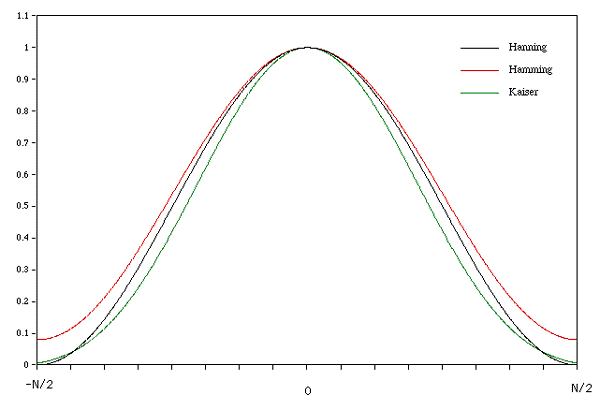Each window has its peculiarities, but in general, we can say a Fourier Transform using Hamming window provides consistent results for the majority of signals.

Back to accuracy, we saw that if you partition a piece of sound too much (a narrow window), spectral leakage is more likely to occur. That's one reason why you can't reduce a window size too much.

Also, it is possible to show - although this is not immediate as you may think - that, when applying the DFT, it is impossible to distinguish two frequencies with difference lower than $$AudioSampleRate/WindowSize$$. Therefore, this parameter, called frequency resolution, gets better as the window size widen. For example, if we choose to sample a 44.1 kHz music with a window size of 1024 it will be impossible to distinguish two frequencies of this music lower than 43.07Hz.

Since this occurs, we must think not in a continuous range of frequencies, but in a discrete world of frequencies, with various bins containing small ranges of them. From the example above, the first bin will contain frequencies that range from 0 to 43.07Hz, the second one will contain frequencies that range from 43.07Hz to 86.14Hz and so on.

By the way, 43.07Hz is a very bad frequency resolution. We must either increase the window size or lower the audio sample rate to get a greater frequency resolution (i.e.: lower frequency range).

What does the DFT give us?

When applying the DFT for a chunk (the small portion of the piece of sound you recorded), you'll end up with a bunch of complex numbers, which will give you information of both amplitude and frequency of that portion. These numbers will give you a general profile of that tiny sound, which will be used to build our fingerprints. Repeat that for the entire piece of sound and you'll obtain even more complex numbers, containing lots of information about your record.

The fact is, those complex numbers contain enough information to create matching criteria with the records on Shazam's database. In the next step we'll study an efficient way to manipulate them and generating our fingerprints.

## Fingerprinting and target zones

For each chunk, we'll have $$\frac {N}{2}$$ bins in the form of complex numbers (remember Nyquist rule?). Therefore, each chunk will contain a bunch of elements with amplitude and frequency information. We can create some rules just to condense the most significative information to our ears. On a sound, take in mind that to the human body, frequency represents the tone and amplitude represents the loudness;

Using a window size of 1024 with a sound containing a sample rate of 11025Hz, for example, we'll have 512 complex numbers for each chunk. We can then filter the numbers that contain less significative frequencies and the lower amplitudes. This will be done by the following:

• Create a array containing 6 empty arrays inside of it. This array will be called 'bands', and will contain the following:

• The first one containing chunk$$[A(0)..A(9)]$$, representing the amplitude of the 10 first bins of frequencies (5.38Hz to 102.18Hz = $$\frac {FrequencyResolution}{2}$$ to $$9FrequencyResolution + \frac {FrequencyResolution}{2}$$;

• The second one containing the elements chunk$$[A(10)..A(19)]$$, representing the next 10 bins of frequencies (107.56Hz to 204.49 Hz);

• The third one containing chunk$$[A(20)..A(39)]$$;

• The fourh one containing chunk$$[A(40)..A(79)]$$;

• The fifth one containing chunk$$[A(80)..A(159)]$$;

• The sixth one containin chunk$$[A(160)..A(511)]$$;

• Keep the greatest amplitude value with of each inner array and cut off the rest. Also, make sure to store the index of those amplitudes on a separate array;

• Take the amplitudes average;

• On the index array, just keep the indexes whose amplitudes are above that average.

Notice we are 'aliasing' the chunk with the average value of frequencies contained on it. As we don't know exactly the ones that are inside of it, its the most sensible thing to do.

Also, this weird scale on the first item is because the human ear have logarithmic perception, so we condense higher frequencies together on wider arrays. The following 3 steps assures we just keep the most significant sound.

After that we'll end up with the index array containing up to 6 values. Those indexes contain information of the frequencies whose amplitudes were the greatest of its bands. To obtain their numerical value, just do the multiplication ($$Index+ ½$$$$\times$$($$\frac {SampleRate}{N}$$) and you are good to go.

Notice this process will be done once for each bin, so you'll have an array with up to 6 values for each discrete time period. That given, our goal is now having a frequency x time function. Here is an example of how it will look: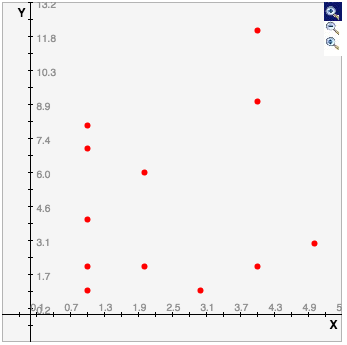5 relevant frequencies on the first chunk, 2 on the second one, 1 on the third one and so on...

Let's recapitulate what we've done so far. We applied the DFT on the original sound. This took us from the time domain to the frequency domain. Graphically, we had a Amplitude x Time plot and went to a Amplitude x Frequency plot. The final step described here took us from the Amplitude x Frequency plot to a Frequency x Time plot. Notice at this point we are getting metrics that are very comparable friendly.

The next step is to make them time efficient.

## Time eficiency

Imagine the scatterplot on the left side is actually a fingerprint of some music on Shazam's database and the one on the right is your recorded sound fingerprint.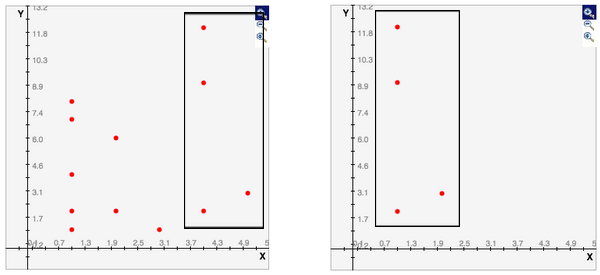Two identical 4-dots set

Eventually, we could make the right plot 'travel' through the left plot and, as the dots overlay, we'd say there's a finegrprint match. This would be very inneficient though, since we had to make lots of comparisons and repeat that for 11+ million different musics.

Let's then, create some rules to make this more efficient.

The base article sugests the concept of target zones. They will help us making those comparisons faster.

The rules that defines a target zone is the following:

• A point on the graph is biunivocally related to a target zone. A target zone's point is called anchor;

• The points will be enumerated from top to bottom, from left to right;

• A point's target zone consists of a set of the five subsequent points of the (point index + 3)th point. If it's impossible to take five points, the set will be composed of the highest number of points available.

• If the rule abose is impossible to apply to a given point, it has no target zone.
#####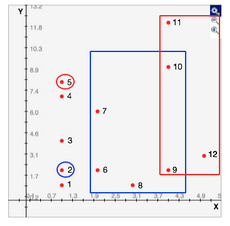Points 2 and 5 and their respective target zones.

Then, we'll make associations between the anchor and each one of its target zone points. Therefore, each anchor will have at max 5 associations. They will be done both on server side, for all those 11+ million musics points, and on the client side, on the piece of sound you recorded. These associations will have the form 'key'=>'value' (a hashtable!).

On both server and client side, each key of a association will correnspond to an array containing the following information:

$$[f(point), f(anchor), \Delta t(point,anchor)]$$, where $$f$$ denotes frequency and $$\Delta t$$ denotes time interval

Notice that we used $$\Delta t$$ and not an absolute time as one of the identifiers. This assures our search will be time independent.

For the values, although, they are going to be slightly different. On server side, it will be an array containing the following:

$$[t(anchor), SongId]$$, where $$t$$denotes the absolute time and $$SongId$$ denotes the Id of the music on the database.

On the client side, it will just have $$t(anchor)$$.

So our associations will be:

On the server side$$[f(point), f(anchor), \Delta t(point,anchor)] => [t(anchor), SongId]$$

On client side$$[f(point), f(anchor), \Delta t(point,anchor)] => t(anchor)$$

Let's take an example:

Supposing the scatterplot above is from Michael Jackson's 'Beat It' and its ID on Shazam's database is 2243 (just a guess), the associations for point 2 are:

• $$[2, 2, 1] => [1, 2243]$$
• $$[6, 2, 1] => [1, 2243]$$
• $$[1, 2, 2] => [1, 2243]$$

• $$[2, 2, 3] => [1, 2243]$$

• $$[9, 2, 4] => [1, 2243]$$

Just take in mind all those frequencies and times on these associations are fictitional. 1~9Hz are really low frequencies that human ear can't even notice. In practice, those values range from 20Hz to 20kHz.

1s time steps are also too big. We generally work with steps of 0.064~0.256 seconds.

All associations from every point corresponds a song fingerprint. We finally got into them!

Notice how, on the keys, we are identifying pairs of frequencies (from the point relatively to the anchor) with their respect distance in time instead of taking the individual points with their absolute time. This makes our associations much more unique, which enhances our search while generating less ‘fake positives’.

If we consider a step of 0.128s and an average of 3 significant frequencies per time unit, a 3 minute song will have a fingerprint with about 27000 associations. This may sound big, but we'll see this its very efficient and much faster than the 'sweeping method'.

## Matching criteria

As we record a piece of (unknown) music, most of the keys from the record are going to be the very same from our database fingerprint. If the keys on the database are indexed, we just need to fetch all the ids containing that key. Do that for every key in the record and you'll end up with a list of music candidates for that record.

Obviously, the music with most key matches are the most likely to be the recorded one. Although, this will work for lots of records, it's not certain that this will always happen. For that reason, is sensible that we think on some scoring methods to optimize our music match.

## Scoring methods

So far, we have 95% of our job done. But notice there’s still an information on our fingerprints we didn’t use. On both the client side and server side fingerprints use the absolute time was kept untouched​. Let’s see how we can take advantage from this number.

Remember our first statement for target zones:

• A point on the graph is biunivocally related to a target zone.

That means an association key is very unique inside a song’s fingerprint. Although it’s possible for a song to contain the exact same key inside its fingerprint with a different value associated to it, this won’t happen very often. Therefore, a pair of different associations are very likely to have an equal time distance (i.e.: the absolute time of the first association subtracted from the apsolute time from the second association) on both the cellphone generated fingerprint and on Shazam’s fingerprint.

Notice we do two time related comparions: one between point and anchor - the third value on the key array - which will appear several time in different associations, but on a trio becomes very unique - and another between two different associations, using their absolute time - which is even more unique. This way we are creating a very powerful filter for fake positives.

Let’s then create a simple scoring criteria:

• For each association match between the record generated fingerprint and Shazam’s database fingerprint, we score a $$(+1)$$ for the referred music;

• For each pair of matched associations, take the time distance between them (one for the association pair on the cellphone record and one for the association pair on Shazam’s DB). If they are equal, we score a $$(+1)$$. If they are different, we score a $$(-1)$$.

• For the scoring process above, remember to ‘walk’ through pairs of associations. For example, if we got a match for $$([1, 2, 3], [2, 2, 1], [3, 4, 3], [1, 1, 1], [2, 1, 2])$$, you could take [1, 2, 3] and [2, 2, 1], then [2, 2, 1] and [3, 4, 3], then [3, 4, 3] and [1, 1, 1] and so on. Avoid mantaining the same key while changing the second one, because the static key could eventually be a fake positive. This would end up scoring multiple $$(-1)s$$ and ruinning our method.

• For each streak like the one above, score $$(+1)$$;

Remember time is quantized when windowing the wave, so the second comparison is very safe. Also, we can always change those methods to fit our needs and get better results.

## Optimizing our fingerprinting

Before start coding, we are going to take a look at some optimizations we can do to increase performance when searching on Shazam's database.

Downsampling

Before generating our fingerprints (both on server and client sides), it's a good idea to artificially reduce the sampling rate from the audio records. This will allow us to use a steady frequency resolution as we reduce the numbers of associations per fingerprint. As a bonus it will take less time to upload you record to Shazam's servers and it will consume less memory on your smartphone.

As an example, we can downsample a 44.1kHz music to 11kHz, mantaining the sample rate to increase the frequency resolution by 4 times its original value. Although these alterations affect dramatically the sound quality, the dominating frequency keeps the same and the fingerprints can be generated normally. To do this, we just take the average of each set of 4 amplitudes before creating the bands array, instead of taking them all.

An alternative to the DFT: The Cooley-Tukey algorithm

Let's get back to the DFT algorithm:Suppose we chose a 1024 window size to apply a DFT on a 11025Hz music with duration of 3 minutes. We'll have then a (1024/11025) = 0.093s time interval between each chunk, which gives us (180/0.093) = 1936 chunks.

For each bin, we'll do (1024-1) summations and 1024 multiplications, totalizing 2047 operations per bin. Do that 1024 times (for each bin in the window). Multiply that value by 1936. You'll end up with more than 4 billion operations per music.

Even with a fast home PC, doing this for your entire 5000 music iPod library would take some days. And this doesn't seem to be very efficient…

Hopefully, there's a faster way to do it, called the Cooley-Tukey method, or simply the Fast-Fourier Transform (or FFT). It takes advantage of the periodicity of sines and cosines to calculate recursively complex values so they can be reused on further calculations.

There's no need to take this so deep. This will take some math to understand, but if you want to skip this part, go ahead. Just take in mind there's an optimal way to calculate the DFT and we will be using it for coding later.

First we will split the original DFT into 2 parts: one containing just the odd components and one containing the even ones. Let's start with the even part.

To take the even values of the summation, just let $$k = 2m$$. As we also want 0 to be the lower bound of summation (just to keep the notation), we keep the index of summation $$m$$ and modify the upper bound of summation instead to $$(\frac {N}{2} - 1)$$. Thus, we get: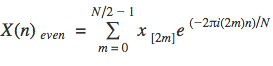For the odd values, let $$k = 2m + 1$$. We get: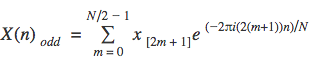This way,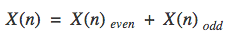If we strategically put $$e^{-2 \pi i n/N}$$ (from now on we'll call it 'twizzle factor') in evidence on $$X(n)_{odd}$$, we get: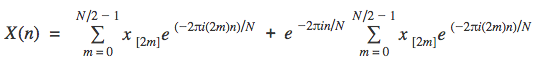As we give the first summation the alias $$\bar X(n)_{even}$$ and the second one $$\bar X(n)_{odd}$$, we'll end up with: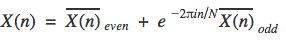As of the periodicity of the FFT: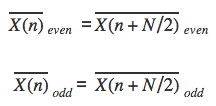Back to the twizzle factor, just by applying the distrubutive property we can see that: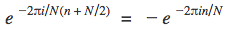Thus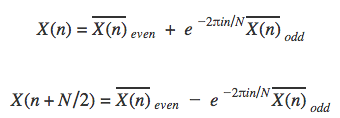The power of this method lies on these two expressions.

Also, for simplicity purposes, let's also define another function $$x:$$

$$F(n) = \begin{cases} \bar X(n/2) & \quad \text{if } n \text{ is even}\\ \bar X((n+1)/2) & \quad \text{if } n \text{ is odd}\\ \end{cases}$$

And supposing window size is N = 8: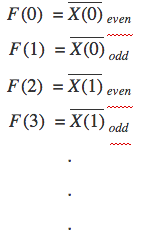Now $$X(0)$$ can be written on a different way: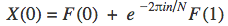Notice how F is dependent on $$\bar X(n)_{even}$$ and on $$\bar X(n)_{odd}$$ Remember that both of them contains half of the operations of the original DFT.

Also notice how we must calculate $$X(0),X(1),..., X(8)$$to obtain the full spectrum.

Now take a look at the highlighted expression above. If we take, let's say$$X(4)$$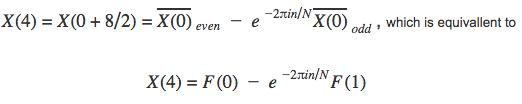Both $$X(0)$$and $$X(4)$$ use $$F(0)$$, $$F(1)$$ and the same twizzle factor. That means we can get two DFTs at once with the same calculations. Those also contain half of the operations of the original DFT! Take a look at the diagram below: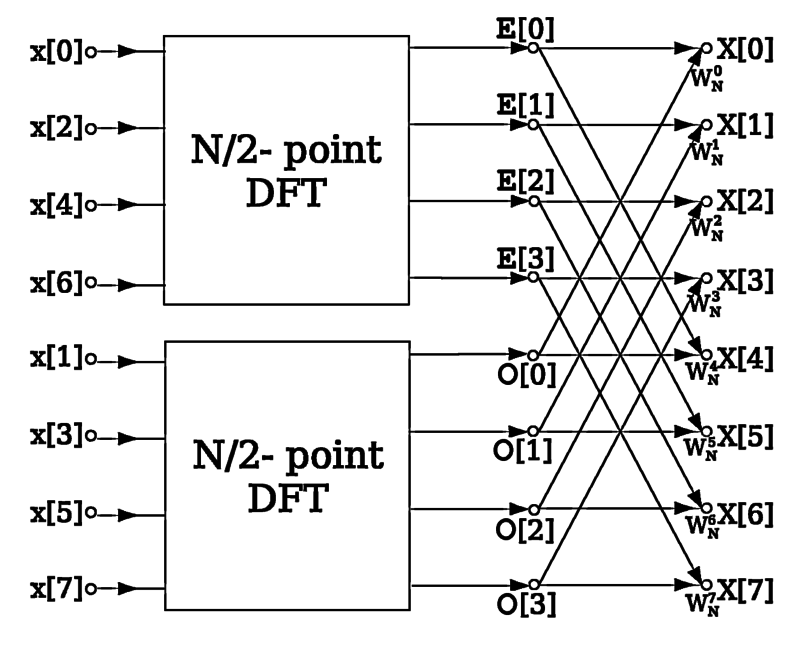By its operation schema, this process is also known as 'Butterfly multiplication'

This method has time complexity of O(N*log(N)) and for big numbers will be lots faster than the original FFT.

Phew, that was a lot of information! Some things must have remained unclear, but everything will make sense when we see a practical application. For the moment, congrats for the patience reaching the end of this arcticle :)

Unsupervised learning shows which senators vote alike

On this article, I get into details of how to write a program capable of grouping Brazilian senators according to their votes. All the way from getting the necessary info, going through some code and math, and finally getting to the Senate's inner circles.

## Introduction

Brazilian's legislative scenery is complex and varies now and then. Basic comprehension of how our representatives behave is usually challenging even to the most well-informed of us. To the ordinary citizen, this information may not arrive, and if it does, it's usually uninterpretable or too prepared.

Luckily, law enforces our government's transparency and accountability; that valuable information can be found on online portals like the one about the Senate. Don't get too excited, though, as information access is another right we've been struggling to wholly get in Brazil.

I decided to start this activity during internship at Infosimples in order to extract impartial yet meaningful conclusions from politicians' votes. It was worth the effort, since it put under test some concepts seen at Udacity's Intro to Artificial Intelligence (well, it also brought up curious readers like you! Leave some feedback later).

What is Data Matching?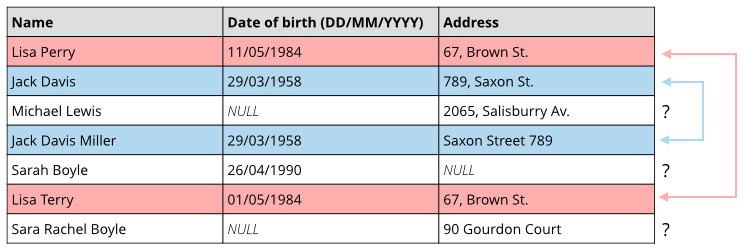Data Matching is the task of finding records that refer to the same entity. Normally, these records come from multiple data sets and have no common entity identifiers, but data matching techniques can also be used to detect duplicate records within a single database.

Data Matching is also known as Record Linkage, Object Identification or Entity Resolution.

Identifying and matching records across multiple data sets is a very challenging task for many reasons. First of all, the records usually have no attribute that makes it straightforward to identify the ones that refer to the same entity, so it is necessary to analyze attributes that provide partial identification, such as names and dates of birth (for people) or title and brands (for products). Because of that, data matching algorithms are very sensitive to data quality, which makes it necessary to pre-process the data being linked in order to ensure a minimal quality standard, at least for the key identifier attributes.

Semantic Analytics with Google Cloud Dataflow (webinar)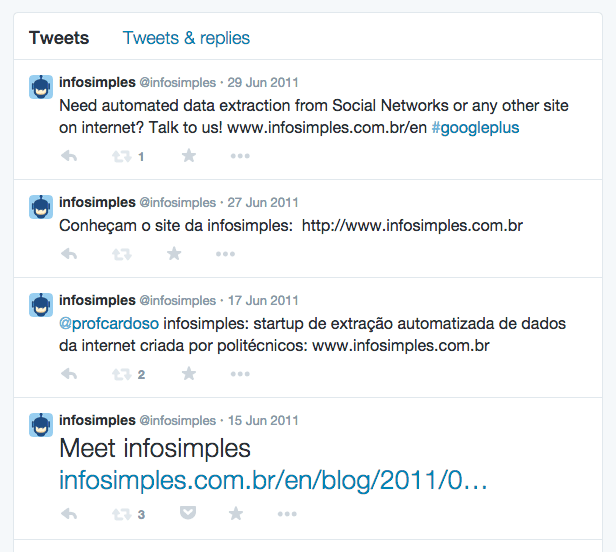September 18, thursday, 9h AM PST

Google is working to provide a new service called Google Cloud Dataflow. It will enable large scale processing of realt-time streaming data, like tweets, activities or events. It may be offered integrated with other Google Cloud services, like Cloud Storage, Big Query and Pub/Sub.

Eric Schmidt, Solutions Architect at Google, will present Google Cloud Dataflow and share how he analyzed the sentiment of millions of tweets streaming real-time from the World Cup in order to track fan response to events happening on the field. He'll show how he calculated average sentiment per team and per match, correlated sentiment with keywords of turning points in the games, and tied it all together with a timeline visualization that lets you track how global fans feel throughout the match.

You can register to the event through the link:

Data engineering over the Titanic disaster

In this article we are going to participate in a challenge of Data Science proposed by Kaggle. This challenge consists in analizing data of passengers from Titanic and build predictions about their fate on the tragic night of the accident.

One of the requirements is the environment to be installed in your machine. I suggest you download RStudio, wich is an IDE that greatly speeds things up and helps a lot, specially with variables and graphics. Another choice is to download only the basic environment (mirrors found here: http://cran.r-project.org/mirrors.html).

There is another pre-requisite we must meet before begin analizing the data: the data itself. Each Kaggle challenge has its own page, ours is www.kaggle.com/c/titanic-gettingStarted. Click on 'Data' and download the files 'train.csv' and 'test.csv'. You'll probably be asked to login before download starts.

The file 'train.csv' will be used as as training set. Random trees are supervisioned learning algorithms, i.e. they need to be fed the expected output along with the input data, so we'll be using the column 'Survived' inside that file as the output when training our model. After our prediciton model is done 'test.csv' will be used to test it.

Now roll up your sleeves and let's get to work!

Deep Learning Webinar promoted by NVIDIA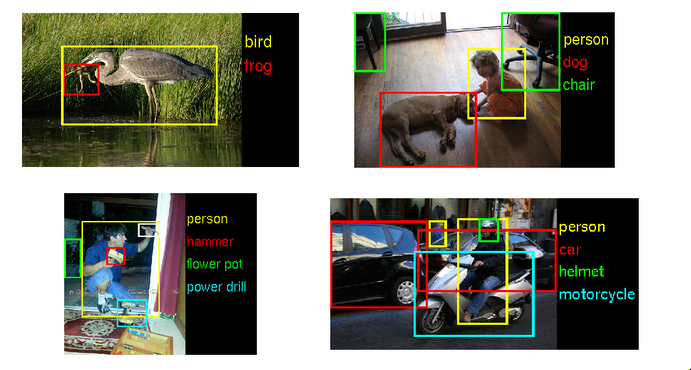On September 24, 2014 09:00 AM PST, Yann LeCun will present a free webinar about Deep Learning, specially about Convolutional Neural Networks. The event is promoted by NVIDIA, which recently claimed they will be making Deep Learning applications easier to program with their GPUs.

Register to the event in the link below:

http://info.nvidianews.com/GTCExpressWebinarSeptember242014.html

Overview of Neural Networks

Overview

The idea of neural network algorithm came as an attempt to mimic the brain and its amazing ability to learn. Although it is a relatively old idea (emerged around the years 80-90), nowadays neural networks is considered the state of the art in many applications.

Neural network algorithms are based on the hypothesis that the brain has only one algorithm that can learn all features of the body, i.e., any area of the brain could learn to see or hear if it received the appropriate stimulus.

Representation

In the brain, each neuron receives nerve impulses through dendrites, performs some "calculation" in cell body and transmits the response via another nerve impulse using the axon. A neural network algorithm copies this system, as shown in Figures 1 and 2 below.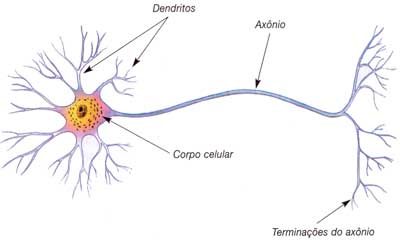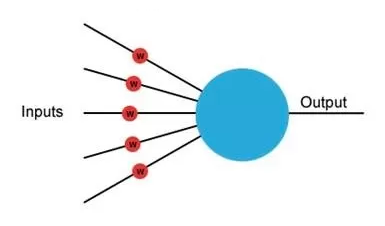##### Figures 1 and 2 - representation of a neuron (left) and a neural network unit (right).

In this type of algorithm, several "neurons" are interconnected to form a network. This network consists of three types of layers, known as input layer, output layer and hidden layers, as shown in Figure 3 below.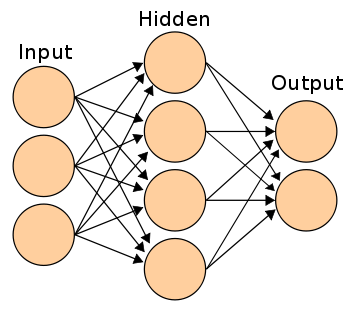##### Figure 3 - representation of a neural network.

The input layer receives the data and the output layers outputs the response. The hidden layers are responsible for some intermediate calculation that helps the network to find the final answer. In more complex networks, one can use multiple hidden layers between the input and the output layers. The number of neurons in each layer depends on the amount of input data and the type of problem being solved. For example, if the algorithm was designed to determine whether or not a patient has a specific disease, the input layer has as many neurons as the number of features in the input data and the output layer has only one neuron.

Artificial Intelligence for Tic-Tac-Toe

The use of reinforcement learning to program the artificial intelligence of games probably has as one of its most impressive achievements the development of a program that could play backgammon at a professional level by Gary Tesauro from IBM during the 90s. The first attempt was to build a model of the many states of the game based on a collection of examples and try to generalize from those using supervised learning. Besides being a tedious job for the researchers to enumerate hundreds of different states, the program was performing poorly.

Using a different approach, this time with reinforcement learning, the developers made two versions of their game play against each other. At the end of each game, the winning one received a positive reward and the losing one a negative. After 200.000 matches, the agent was able to play at the level of some of the best players in the world, without the need of any kind of professional experience or describing the more than 1 trillion possible states of the game.

In an attempt to recreate the experiment with a simpler game, the idea was to develop an intelligent agent for Tic-Tac-Toe, without the need to write an algorithm with the best strategies or enumerating all the possible states of the game.

String Segmentation using Machine Learning

Artificial Intelligence has brought lots of improvements to problems related with language processing, especially in the field of language translation. Other examples include suggestions to correct misspelled words or even speech recognition.

To test a simple algorithm that works with elements of a language, the developed program had the objective of being able to split a string without spaces into the words that compose that string. For instance, receiving the string "thisishowitshouldwork" should result in "this is how it should work".

The problem consists in being able to identify words, since it's impossible to list all the known english words and there is no model that can correctly generalize the structure of every word.

The approach was to use supervised learning and a database of texts as training data so that the agent could start to identify the different words that exist in the english language. By utilizing texts instead of just a list of thousands of different words, it's possible to identify the frequency in which every word tend to appear. Knowing the probability of each word showing up, the agent should be able to calculate the most probable segmentation of every string.

It's viable to say that the probability of a certain segmentation depends on the probabilities of each word obtained by that segmentation. By considering the words that show up before the one we are analysing, the results could be even more accurate but, with the relatively small quantity of training data available, it was only taken in consideration the individual probability of each word.

Another point that has to be taken into consideration is how to deal with words absent from the training data. It's impossible to say that any training database contain every known word, no matter how big it its. This issue was adressed by using Laplacian Smoothing to avoid unknown probabilities to being equal to zero. At last, the calculations should be normalized so it's possible to compare segmentations between a different number of words.

The probability of a single word can be expressed by: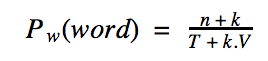where n is the number of times the word appeared on the training data, T the total of analysed words, k is a constant and V is the estimated total number of words on the english vocabulary. On this problem it was used k = 1 and V = 2,000,000.

The probability of a segmentation can be expressed by: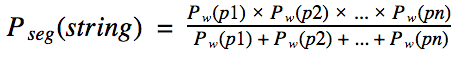where p1, p2, ... pn are the possible words obtained on a segmentation.

Knowing how the calculations should be done, it's necessary to deal with the problem of hardware limitations. A string with n characters has 2n-1 ways of being split, which would result in over one million calculations just to identify the best segmentation of the string "thisishowitshouldwork".

To deal with this problem, it's possible to adjust the algorithm to always split the string in two pieces, f (first part) and r (rest). If the probability of f is X times bigger than r's, the agent has likely found a good segmentation in f, and should try to segment r as well.